# Saxon Calculus: Computation of Derivatives Chapter Exam

Exam Instructions:

Choose your answers to the questions and click 'Next' to see the next set of questions. You can skip questions if you would like and come back to them later with the yellow "Go To First Skipped Question" button. When you have completed the practice exam, a green submit button will appear. Click it to see your results. Good luck!

### Page 1

#### Question 1 1. Integrate the following.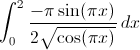#### Question 2 2. Find the derivative df/dt.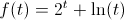#### Question 3 3. Find the derivative of f(x).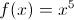#### Question 4 4. Evaluate.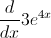#### Question 5 5. Integrate the following.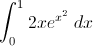### Page 2

#### Question 6 6. Find the jerk of the function shown below: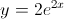#### Question 7 7. Find the derivative of f(x).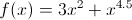#### Question 8 8. Given the derivative of a function (ƒ′(x)), which of the following could be ƒ(x)?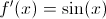#### Question 9 9. Find the derivative df/dx.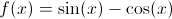#### Question 10 10. Evaluate the following second order derivative.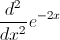### Page 3

#### Question 11 11. Find dy/dx given the following equation: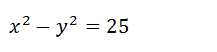#### Question 12 12. Find f'(x).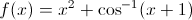#### Question 13 13. Find the derivative df/dt, given the function f(t).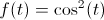#### Question 14 14. Evaluate the derivative.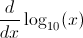#### Question 15 15. Evaluate.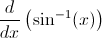### Page 4

#### Question 16 16. Evaluate the following.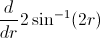#### Question 17 17. Integrate the following.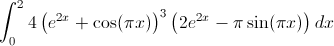#### Question 18 18. Find the second derivative of f(t) shown below.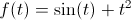#### Question 19 19. Find the derivative of f(x).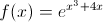#### Question 20 20. Find the derivative of y(t).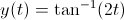### Page 5

#### Question 21 21. Find f'(x) for the following function.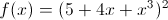#### Question 22 22. Find the derivative of f(x).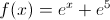#### Question 23 23. Find the second derivative of f(x) shown below: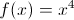#### Question 24 24. Integrate the following.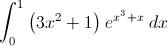#### Question 25 25. What is the highest-order derivative of f(x) that is NOT zero?### Page 6

#### Question 26 26. Find dy/dx of the given equation: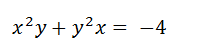#### Question 27 27. Integrate the following.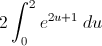#### Question 29 29. Find the derivative of f(x).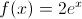#### Question 30 30. Find dy/dx given the following implicit equation: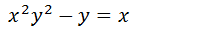#### Saxon Calculus: Computation of Derivatives Chapter Exam Instructions

Choose your answers to the questions and click 'Next' to see the next set of questions. You can skip questions if you would like and come back to them later with the yellow "Go To First Skipped Question" button. When you have completed the practice exam, a green submit button will appear. Click it to see your results. Good luck!

Support